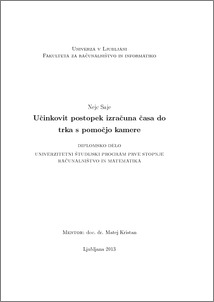# An efficient camera-based approach for estimating time-to-collision

Nejc Saje (2013) An efficient camera-based approach for estimating time-to-collision. EngD thesis.Preview
PDF

## Abstract

The primary objective of this thesis was the implementation of an algorithm for calculating time to contact with an object the camera is approaching. Time to contact is calculated using information about the change of object's size over time. This information is extracted from a sparse motion field which is calculated using a fast keypoint detector and an efficient keypoint descriptor. Correspondences are filtered using epipolar geometry. Because the data is noisy, the focus of expansion is calculated as the intersection of lines that run through the vectors of the motion field. Depending on the amount of keypoints, the size of the region surrounding the focus of expansion is chosen and the global time to contact is calculated using the points inside that region. The image is divided in regions and the probability of collision is calculated using time to contact calculations, the variance of calculations inside the region and the number of points in the region. The implemented algorithm was tested on image sequences acquired in controlled environments.

Item Type: Thesis (EngD thesis)
Keywords: time to contact, sparse motion field, keypoints, epipolar geometry, computer vision, camera
Number of Pages: 55
Language of Content: Slovenian
Mentor / Comentors:
Name and SurnameIDFunction
doc. dr. Matej Kristan4053Mentor